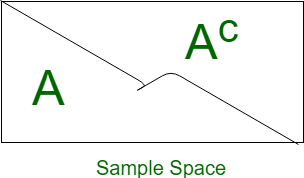Open in App
Not now

# GRE Data Analysis | Probability

• Last Updated : 25 Jan, 2023

Probability is a numerical representation of the chance of occurrence of a particular event. Here the event is the word used to describe any particular set of the outcome.For Example, when a coin is tossed the total number of possible outcomes are 2 i.e {Head, Tail}. And the probability of getting head, P(Head) is 1/2. since the number of success for getting head is 1.

• Sample space: Total number of possible outcome for a event is known as its sample space. It is generally denoted by S.
• Equally likely: Two events are equally likely if they have equal probability of occurrence.
• Event: Event can also be described as the subset of sample space.
• Trail: It is an action which results in one or many outcomes.
• Mutually Exclusive: Two events are said to be mutually exclusive when both can not occur at same time.
• Independent Event: Two events lets say A and B, are independent of each other when occurrence of one does not effect occurrence of other. When two events, A and B, are independent, the probability of both occurring is:
P(A and B) or P(A ∩ B) = P(A)·P(B)
1. 0 ≥ P(E) ≤ 1, i.e Probability of an event is always greater than or equal to zero and less than or equal to one.
2. P(S) = 1, Here S represents sample space. The probability of occurrence of atleast one event of sample space is 1. If the probability of event is 1 then it is certain that the event will occur.
3. P(A ∪ B) = P(A) + P(B), where A and B are two mutually exclusive events, i.e P(A ∩ B) = φ

1. P(Ac)= 1 – P(A), where P(Ac) is complement of P(A).2. When two events, A and B, are not-mutually exclusive, the probability that A or B is:
P(A or B) = P(A) + P(B) - P(A and B)

Example-1: Tokens numbered 1 to 20 are mixed up and then a token is drawn at random. What is the probability that the token drawn has a number which is a multiple of 3 or 5? Solution: Total possible outcome,

S = {1, 2, 3, 4, 5, ...20}

The favorable outcome, which is multiple of 3 or 5 is

E = {3, 6, 9, 12, 15, 18, 5, 10, 20}

Then,

P(E) = n(E)/ n(S)

where n(E) represents number of favorable outcome

P(E) = 9/20

Example-2: An Urn contains 2 red, 3 green, and 5 blue balls. Two balls are drawn at random. What is the probability that none of the balls drawn is blue? Solution:

Total number of ball = 10, Number of blue ball = 5
P(Blue) = Number of blue balls / total number of balls
P(Blue) =  5/10 = 1/2

P(not blue) = 1 - P(Blue)
P(not blue) = 1- 1/2 = 1/2 

Example-3: Find the probability of getting sum more that 7, when we simultaneously throw a pair of dice. Solution: Total number of outcome,

= 62 = 36

Number of favorable outcome,

= {(2, 6)
(3, 5) (3, 6)
(4, 4) (4, 5) (4, 6)
(5, 3) (5, 4), (5, 5) (5, 6)
(6, 2) (6, 3) (6, 4) (6, 5) (6, 6)} = 15

P(sum more than 7) = 15/36 = 5/12 

Example-4: Find the probability of getting two consecutive Heads when three coins are tossed simultaneously. Solution:

Total number of outcome,
= 23 = 8

These are,
{(HHH), (HTH), (HHT), (HTT), (THH), (THT), (TTH), (TTT)}

Number of favorable outcome,
= {(HHH), (HHT), (THH)} = 3

P(getting two consecutive heads) = 3/8  
My Personal Notes arrow_drop_up
Related Articles